# Revision Notes For CBSE Class 10 Maths Chapter 12 Areas Related to Circles

When we talk about the chapter “areas related to circles” in class 10 maths textbook, students will need to study the concepts of the perimeter (circumference) and area of a circle. The chapter also has an objective of preparing students to apply their theoretical or practical understanding in finding the areas of circles and its parts known as segment and sector as well as finding the areas of some combinations of plane figures. To help students get a good hold of the concepts and get familiar with some of the methods of solving problems, free CBSE revision notes for class 10 maths chapter 12 – areas related to circles is provided here. The notes contain clear-cut explanations that will boost student’s knowledge about the topics and at the same time help them get better at solving all the questions given in this chapter. These notes also act as a great study tool for last-minute revisions.

Get a glimpse of class 10 maths chapter 12 CBSE revision notes PDF below.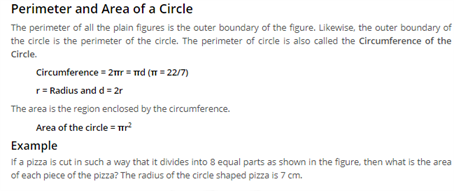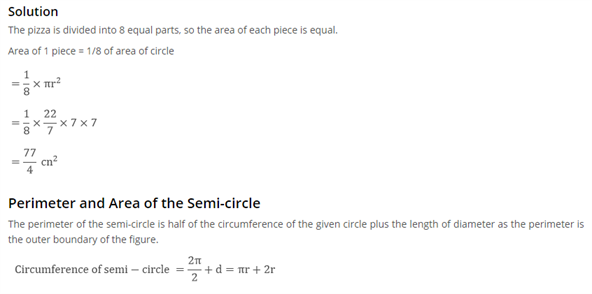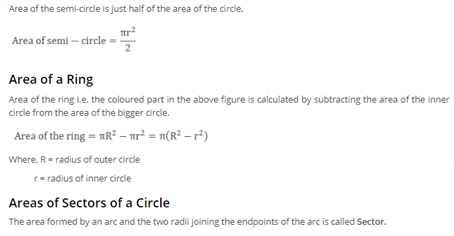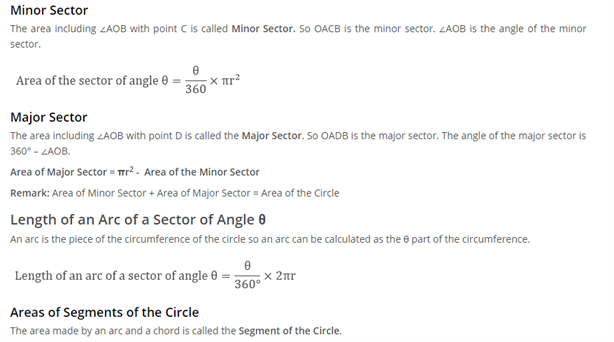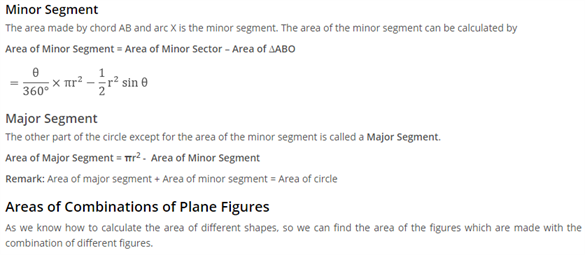Access CBSE Class 10 Maths Sample Papers Here.

Access NCERT Class 10 Maths Book Here.